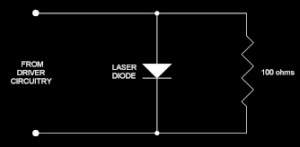ESD polarity terminology used on this web site

The term “positive-ESD” is used to mean electrostatic discharge (ESD) whose voltage polarity would tend to forward-bias a laser diode. “Negative-ESD,” means ESD whose voltage polarity would tend to reverse-bias a laser diode.

 Review of ESD approaches START/PREV CURRENT NEXT END Simple resistor

Review of ESD approaches

# Simple resistor used as an ESD protection meansThe figure to the right shows one of the ESD protection means known to be employed for protecting laser diodes. In this scheme, a resistor is connected directly across the laser diode terminals, within the head, and with the resistance being typically around 100 ohms.

Using the human body model shown in the figure as a guide, it is easy to see that this protection means will not be effective. As discussed above, for a 15,000 volt ESD event, the resistance would need to be less than 44 milliohms, in order to prevent the laser diode voltage from exceeding the maximum reverse bias voltage of 2.0 volts and exceeding a similar forward-bias voltage. If a 100-ohm resistor is used, it would allow (15,000 volts / (330 ohms + 100 ohms)) / 100 ohms = 3488 volts to surge into the laser diode. Since this is far in excess of the typical 2.2-volt lasing threshold or 2.0-volt maximum reverse bias voltage, this would almost surely destroy the laser diode.

Although it might seem that the 100-ohm resistor could simply be replaced with a 44-milliohm resistor, this is not practical, because it would mean that, during operation, far more power would be expended in the operation of the protection resistor than the laser diode itself.